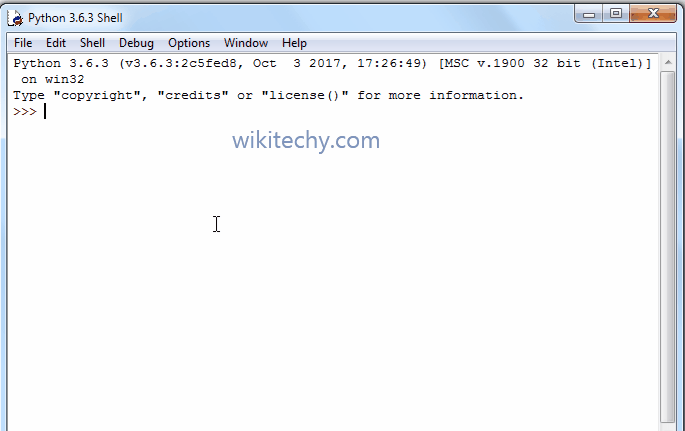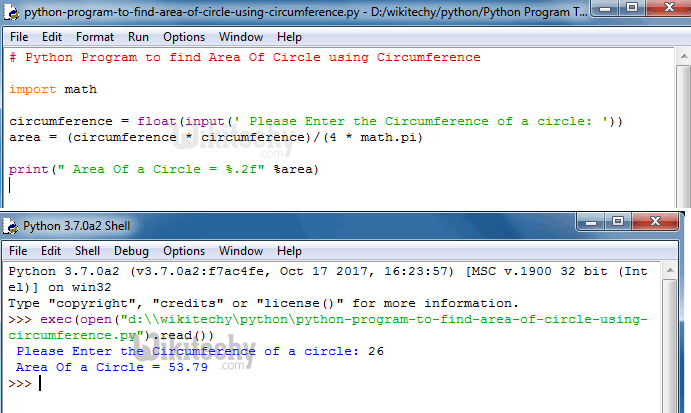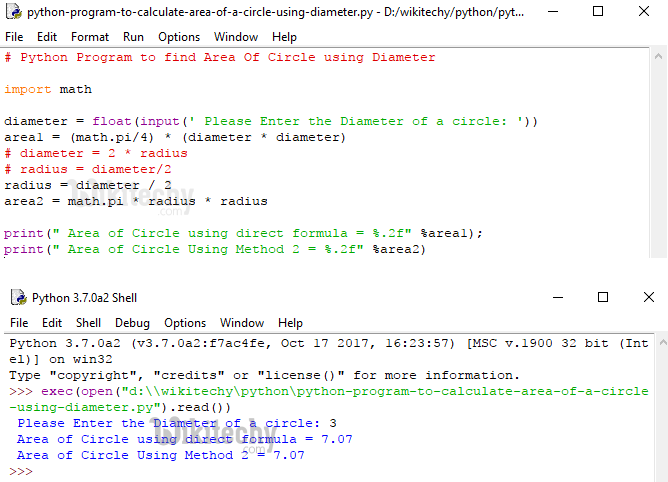# python tutorial - Python Program To find Area Of Circle - learn python - python programming

## Area Of a Circle

• The area of a circle is number of square units inside the circle. Standard formula to calculate the area of a circle is: A=πr².
• How to write python program to find area of circle using radius, circumstance and diameterLearn Python - Python tutorial - Python Program To find Area Of Circle - Python examples - Python programs

## Python Program to find Area Of Circle using Radius

• If we know the radius then we can calculate the area of a circle using formula: A=πr² (Here A is the area of the circle and r is radius).

## Analysis:

• We defined pi as global variable and assigned value as 3.14.
• This program allows user to enter the value of a radius and then it will calculate the area of circle as per the formula.

## OutputLearn Python - Python tutorial - Python Program to find Area Of Circle using Radius - Python examples - Python programs

## Python Program to find Area Of Circle using Circumference

• Distance around the circle is called as circumference. If you know the circumference then we can calculate the area of a circle using formula: A= C²⁄ 4π (Here C is circumference)

## Analysis:

• First, We imported the math library and this supports us to use all the mathematical functions in Python programming.
• In this example we can call the PI value using math.pi
• Next line of code allows user to enter the value of a circumference.
• Using the circumference, this program will calculate the area of circle as per the formula: A= C²⁄ 4π

## OutputLearn Python - Python tutorial - Python Program to find Area Of Circle using Circumference - Python examples - Python programs

## Python Program to Calculate Area Of a Circle using Diameter

• Distance across the circle passing through the center is called as diameter.
• If we know the diameter then we can calculate the area of a circle using formula: A=π/4*D² (D is the diameter)

## Analysis:

• This program allows user to enter the value of a diameter and then it will calculate the area of circle as per the formula we shown above.

We also mentioned other approach.